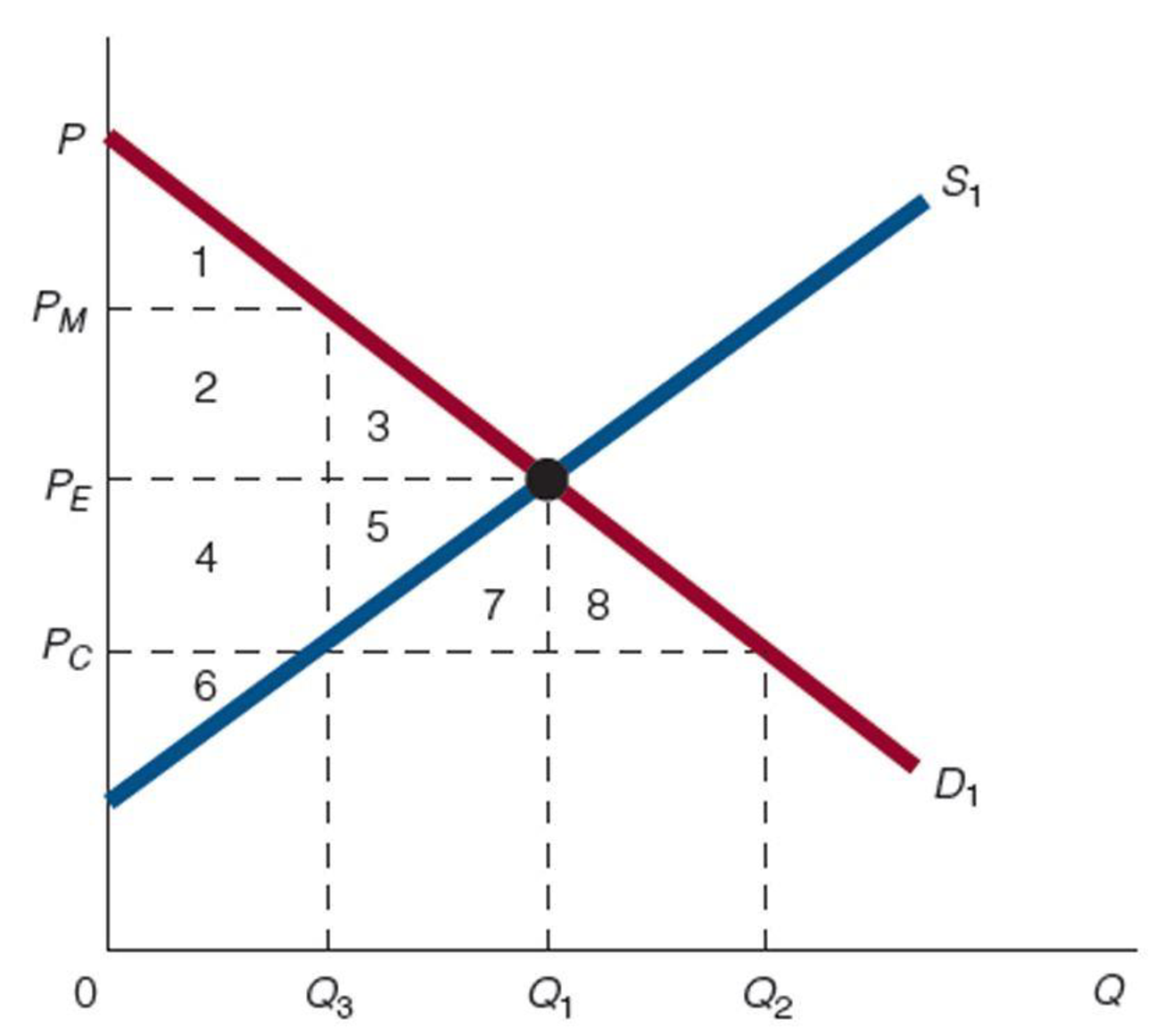Chapter 4, Problem 1WNG

Chapter
Section
Textbook Problem

In the diagram, what areas represent the deadweight loss due to the price ceiling (PC)?To determine

The deadweight loss due to price ceiling.

Explanation

Figure 1 shows the demand curve and supply curve:

The horizontal axis of Figure 1 measures the quantity, and the vertical axis measures the price. The quantity supplied is given by the supply curve S1, and the demand is given by the demand curve D1...

Still sussing out bartleby?

Check out a sample textbook solution.

See a sample solution

The Solution to Your Study Problems

Bartleby provides explanations to thousands of textbook problems written by our experts, many with advanced degrees!

Get Started

Find more solutions based on key concepts